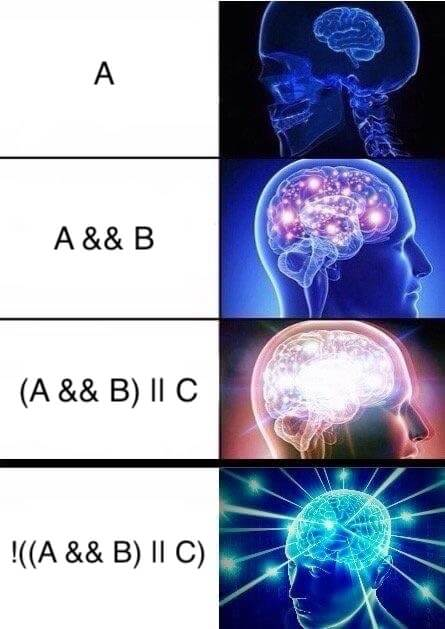# 🔢【程序中的数学】利用德摩根定律简化布尔运算

· 9 分钟阅读

### 一、缘起：一段让人头大的逻辑判断#

if(!((A && B) || C)) {  // do something} else {  // do something}### 二、什么是德摩根定律#

• 命题逻辑里，可以这样表示：
$\neg (P\lor Q)\iff (\neg P)\land (\neg Q)$
$\neg (P\land Q)\iff (\neg P)\lor (\neg Q)$

• 集合里可以这样表示：
$\overline {A\cup B} = \overline {A} \cap \overline {B}$
$\overline {A\cap B} = \overline {A} \cup \overline {B}$

• 布尔代数里可以这样表示：
$\overline {(x \cdot y)} = \overline {x} + \overline {y}$
$\overline {(x+y)} = \overline {x} \cdot \overline {y}$

• 上面的公式还可以用文字描述

• 或者用程序员熟悉的与或非逻辑运算符表示：
!(P || Q) = !P && !Q!(P && Q) = !P || !Q

$P$$Q$$\neg P$$\neg Q$$(P\land Q)$$\neg (P\land Q)$$(\neg P)\lor (\neg Q)$
0011011
0110011
1001011
1100100### 三、解决问题#

!((A && B) || C) == !(A && B) && !C   // 德摩根律== (!A || !B) && !C  // 德摩根律== (!A && !C) || (!B && !C)  // 分配律

(!A && !C) || (!B && !C)== (C && !C) || (!B && !C) // !A 等价于 C（从业务上分析的，不具备普遍意义）== false || (!B && !C)     // C && !C 必定为 false== !B && !C== A && !B  // A 等价于 !C（从业务上分析的）

### 四、化简还有什么招？#

$y$$\overline {y}$
$x$1
$\overline {x}$11